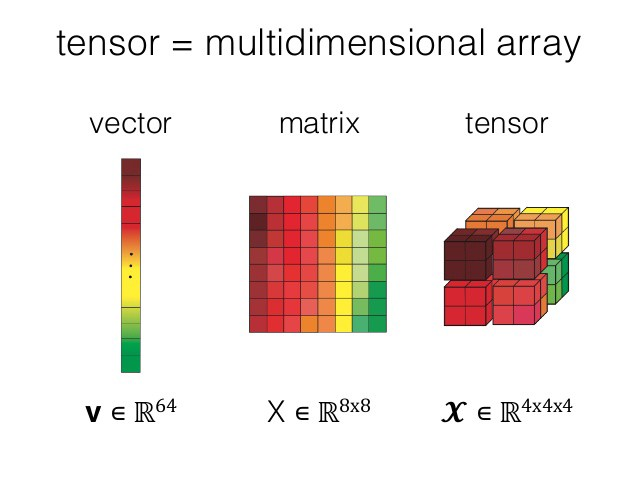# PyTorch 张量(Tensor)介绍## 介绍

1：支持 GPU 运算
2：支持自动求导

• Scalar
• Vector
• Matrix

Scalar 0维张量 标量就是一个数字
Vector 1维张量 向量
Martix 2维张量 矩阵## 初始化

### 1. 直接创建

torch.tensor(data, *, dtype=None, device=None, requires_grad=False, pin_memory=False) → Tensor

data 可以是

• list
• tuple
• scalar### 2. 通过专有的方法

PyTorch 同 Numpy 一样，提供了大量的快速创建数据的辅助方法

ones(*sizes) 全1
zeros(*sizes) 全0
eye(*sizes) 对角为1，其余为0
arange(s,e,step) 从s到e，步长为step
linspace(s,e,steps) 从s到e，均匀分成step份
rand/randn(*sizes) 随机数
normal(mean,std)/uniform(from,to) 正态分布/均匀分布
randperm(m) 随机排列### 3. 通过 tensor### 4. 通过 Numpy## 属性## 运算

### 1. 索引和切片

[行开始:行结束:步长, 列开始:列结束:步长]

• 行开始、列开始不写默认为 0
• 行结束、列结束不写默认为全部
• 步长默认为 1Pending

### 3. View

PyTorch 运行通过 View 来提供不同 Shape 的的数据，View 共享原 tensor 的数据### 4. 广播• 每个 tensor 至少有一维
• 遍历所有的维度，从尾部维度开始，每个对应的维度大小要么相同，要么其中一个是 1，要么其中一个不存在### 5. 连接

torch.cat(inputs, dim=0) → Tensor### 6. 切分

torch.split(tensor, ssplit_size_or_section, dim=0)

split_size_or_sections

• int tensor 会被切分成等大的块，如果最后一块不足，则单独成一块
• list(int) 会被切分成len(ssplit_size_or_section)块，每块大小根据ssplit_size_or_section指定torch.chunk(input, chunks, dim=0) → List of Tensors### 7. 原地修改

PyTorch 的方法带有下划线的`_`的是原地修改，返回修改之后的tensor，而不带下划线的是返回新值，需要注意## 转换

### 1. Tensor to Numpy

``````x = torch.rand(3, 4)
print(x)

y = x.numpy()
print(y)
``````

### 2. Numpy to Tensor

``````x = np.random.rand(3, 4)
print(x)

y = torch.from_numpy(x)
print(y)
``````

## 共享区域

• Tensor 转 Numpy• Numpy 转 Tensor## 参考